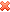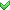178 views

Consider a simple connected undirected graph G which has m vertices and n edges. Which of the following condition always guarantee that after removal of those number of edges graph will be disconnected?

•mn + 2
•• n – 2
• None of the above
| 178 views
0

option B is mC2 -n+2

0

The key point here is :

We know a tree is a minimally connected graph..So if one less the number of edges in a tree is there , then it is always guaranteed that the resultant graph will be disconnected..

So given m vertices , n edges..

Hence no of edges in tree   =  m - 1

Hence one less will guarantee that the graph will be disconnected i.e. = m - 2..

Now initially we have n edges..Let no of edges to be removed  =  x..Hence

n - x =  m - 2

==>    x   =  n - m + 2

selected by